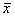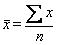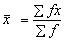## Statistical Averages

Summary Statistics
After the data have been properly checked for its quality, the first and foremost analysis is usually for the descriptive statistics. The general aim is to summarize the data, iron out any peculiarities and perhaps get ideas for a more sophisticated analysis. The data summary may help to suggest a suitable model which in turn suggests an appropriate inferential procedure. The first phase of the analysis will be described as the initial examination of the data or initial data analysis. It has many things in common with explanatory data analysis which includes a variety of graphical and numerical techniques for exploring data. Thus explanatory data analysis is an essential part of nearly every analysis. It provides a reasonably systematic way of digesting and summarizing the data with its exact form naturally varies widely from problem to problem. In general, under initial and exploratory data analysis, the following are given due importance.
Measures of Central Tendency
One of the most important aspects of  describing a distribution is the   central   value   around   which the observations are distributed. Any arithmetical measure which is intended to represent the center or central value of a  set of observations is known as measure of central tendency.
The Arithmetic Mean (or simply Mean)
Suppose that n observations are obtained for a sample from a population. Denote the values of the n observations  by x1, x2.....xnx1   being  the  value  of  the  first  sample observation, x2   that of second observation and  so  on.  The   arithmetic mean or mean or average denoted byis given by
The symbol S ( read as ‘sigma’ ) means sum the individual values x1 ,x2,...,xn  of the variable, X.  Usually the limits of the summations are not written, since it is always understood that the summation is over all n values. Hence we can writeThe above formula enables us to find the mean when values x1, x2 ,....,xn   of  n discrete   observations  are available. Sometimes the data set are given in the form of  a  frequency distribution table then the formula is as follows:
Arithmetic Mean of Grouped Data
Suppose that there are k classes or intervals. Let x1, x2 ,..., xdenote the class mid-points   of   these k  intervals and let f1, f2, ..., fk denotes the corresponding frequencies of these classes.  Then the arithmetic mean
Properties of the arithmetic mean

(a)        The  Sum of the deviations of a set  of  n  observations x1 , x2,..., xn  from their meanis zero.  Let  di as deviation of  xi  fromthen
(b)        If x1 ,x2,...,xn are n observations,is their mean and di = xi - A is the deviation of xi from  a given  number A, then
(c)        If  the  numbers  x1 , x2 ,..., xn  occur  with   the  frequencies  f1 , f2,..., fn  respectively   and   di = xi - A, then
(d)       If in a frequency distribution all the k class  intervals  are  of  the  same  width  c, and  di =  xi - A denote the deviation of  xi from A, where A is the value of a certain mid-point and x1, x2 ,..., xk   are the class mid-points  of the k-classes, then di  = c ui   where ui  = 0, ± 1, ± 2,.....   and
The Median
The  median  of  a set of  n  measurements  or  observations x1 , x2 ,..., xn  is the middle value when the measurements  are arranged  in an array according to their order of  magnitude. If  n is odd, the middle value is the median. If n  is  even, there  are two middle values and the average of these  values is the median. The median is the value which divides the  set of  observations into two equal halves, such that 50% of  the observations  lie  below  the  median  and   50%  above   the median. The median is not affected by the actual values of the observations but rather on their positions.
The Median of Grouped Data
The formula of median of grouped data is as
The Mode
The mode is  the observation  which occurs most  frequently in a set. In grouped data mode is worked out as
The mode can be determined analytically in the case of continuous distribution. For a symmetrical distribution, the mean, median and mode coincide. For a distribution skewed  to the left ( or negatively skewed distribution ), the mean, the median and the mode are in that order (as they appear in  the dictionary ) and for a distribution skewed to the right (  or positively  skewed  distribution) they occur in  the  reverse order, mode, median and mean. There   is   an empirical formula for   a   moderately asymmetrical skewed distribution, it is given by Mean - Mode = 3 (Mean - Median)
The Geometric Mean
There  are  two other averages, the  geometric  mean  and harmonic  mean which are sometimes used. The Geometric  Mean (  GM ) of a set of observations is such that  its  logarithm equals the arithmetic mean of the logarithms of the values of the observations.  GM = (x1  x2..... xn)1/n

log GM = 1/n  (å log xi) or in frequency distribution, log GM = 1/n (å fi log xi)
In case of frequency distribution,
The  geometric mean  can be obtained only if  the  values assumed by the observation  are positive( greater than zero).
Harmonic mean
The Harmonic Mean ( HM ) of a set of observations  is such that its reciprocal is the arithmetic mean of the  reciprocals of the  values of the observation

The harmonic mean is rarely computed for a frequency distribution.
Weighted Mean
If there are n observations, x1, x2, x3,…,xn with corresponding weights w1, w2, w3,…,wn, then the weighted mean is given by,In computing the mean, we take the frequency of a class as its weight.  That isHence, it is a special case of weighted mean. The three means are related by
A.M. ³  G.M. ³  H.M.

Important characteristics of a good average
Since an average is a representative item of a distribution it should possess the following properties :
1. It should take all items into consideration.
2. It should not be affected by extreme values.
3. It should be stable from sample to sample.
4. It should be capable of being used for further statistical analysis.
Mean satisfies all the properties excepting that it is affected by the presence of extreme items. For example, if the items are 5, 6, 7, 7, 8 and 9 then the mean, median and mode are all equal to 7. If the last value is 30 instead of 9, the mean will be 10, whereas median and mode are not changed. Though median and mode are better in this respect they do not satisfy the other properties. Hence mean is the best average among these three.
When to use different averages
The proper average to be used depends upon the nature of the data, nature of the frequency distribution and the purpose.
If the data is qualitative one, only mode can be computed. For example, when we are interested in knowing the typical soil type in a locality or the typical cropping pattern in a region we can use mode. On the other hand, if the data is quantitative one, we can use any one of the averages
If the data is quantitative, then we have to consider the nature of the frequency distribution. When the frequency distribution is skewed (not symmetrical) the median or mode will be proper average. In case of raw data in which extreme values, either small or large, are present, the median or mode is the proper average. In case of a symmetrical distribution either mean or median or mode can be used. However, as seen already, the mean is preferred over the other two.
When we are dealing with rates, speed and prices we use harmonic mean. If we are interested in relative change, as in the case of bacterial growth, cell division etc., geometric mean is the most appropriate average.